# NCERT Solutions For Class 8 Maths Chapter 13 Exercise 13.1 – Direct and Inverse Proportions

NCERT Solutions for Class 8 Maths Chapter 13 Exercise 13.1 – Direct and Inverse , has been designed by the NCERT to test the knowledge of the student on the topic – Direct Proportion

### NCERT Solutions For Class 8 Maths Chapter 13 Exercise 13.1 – Direct and Inverse Proportions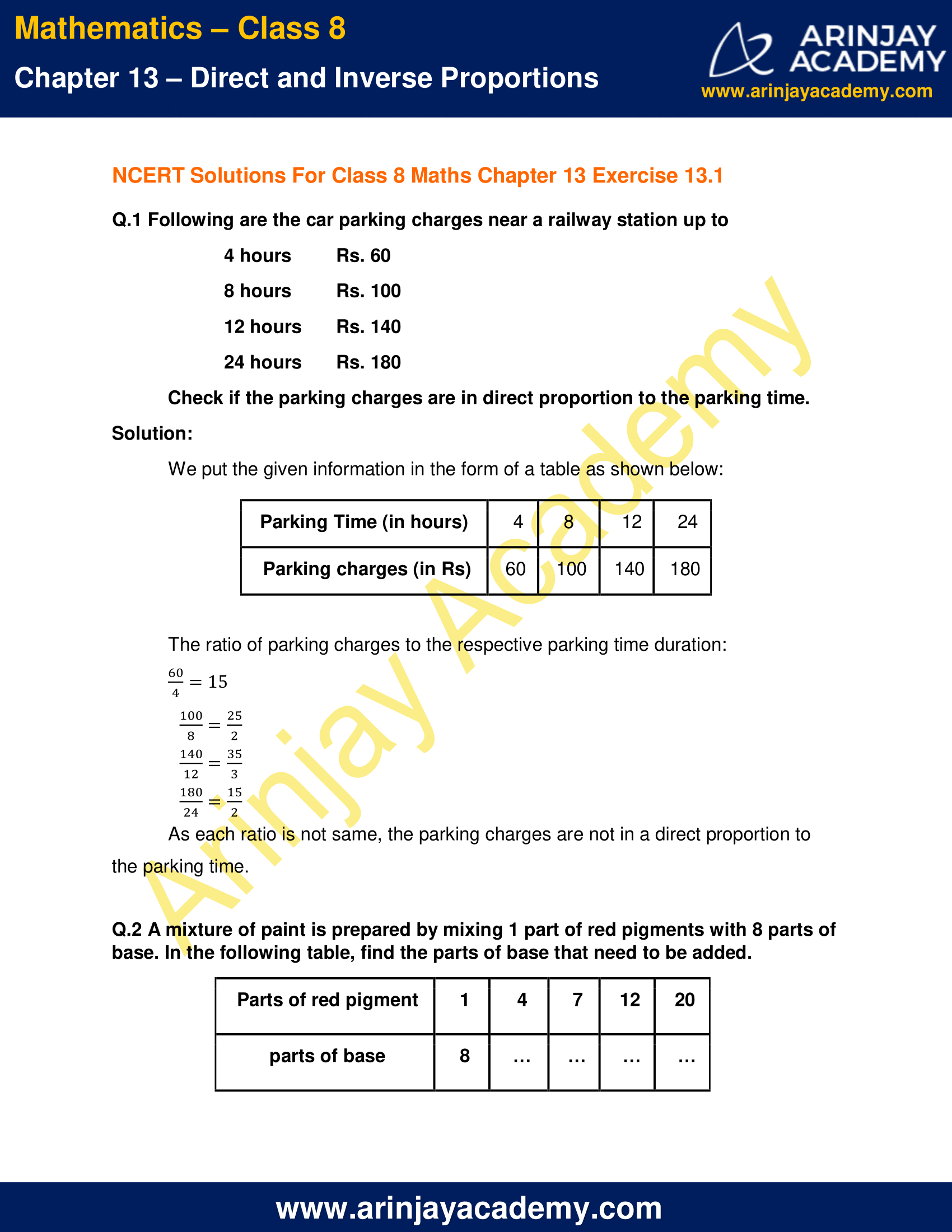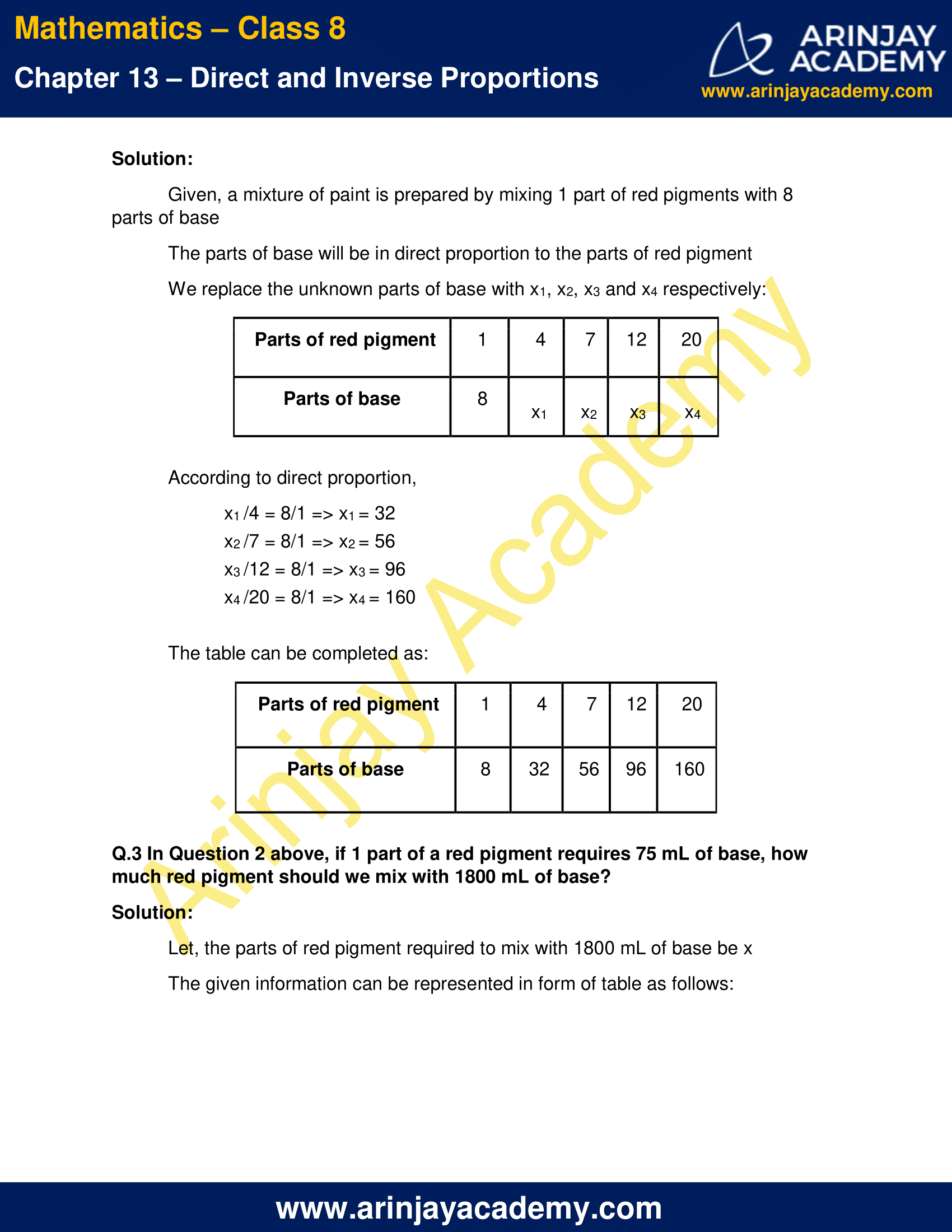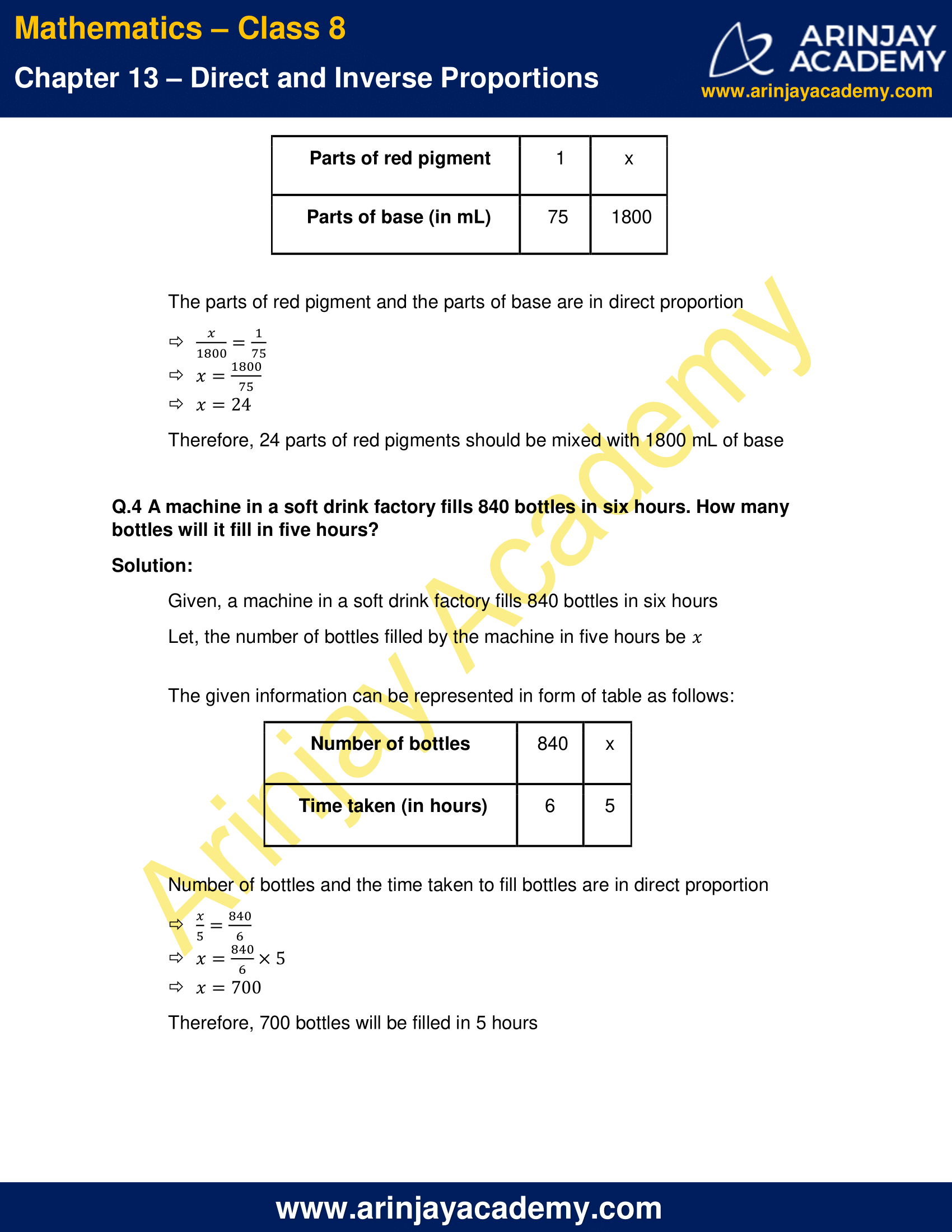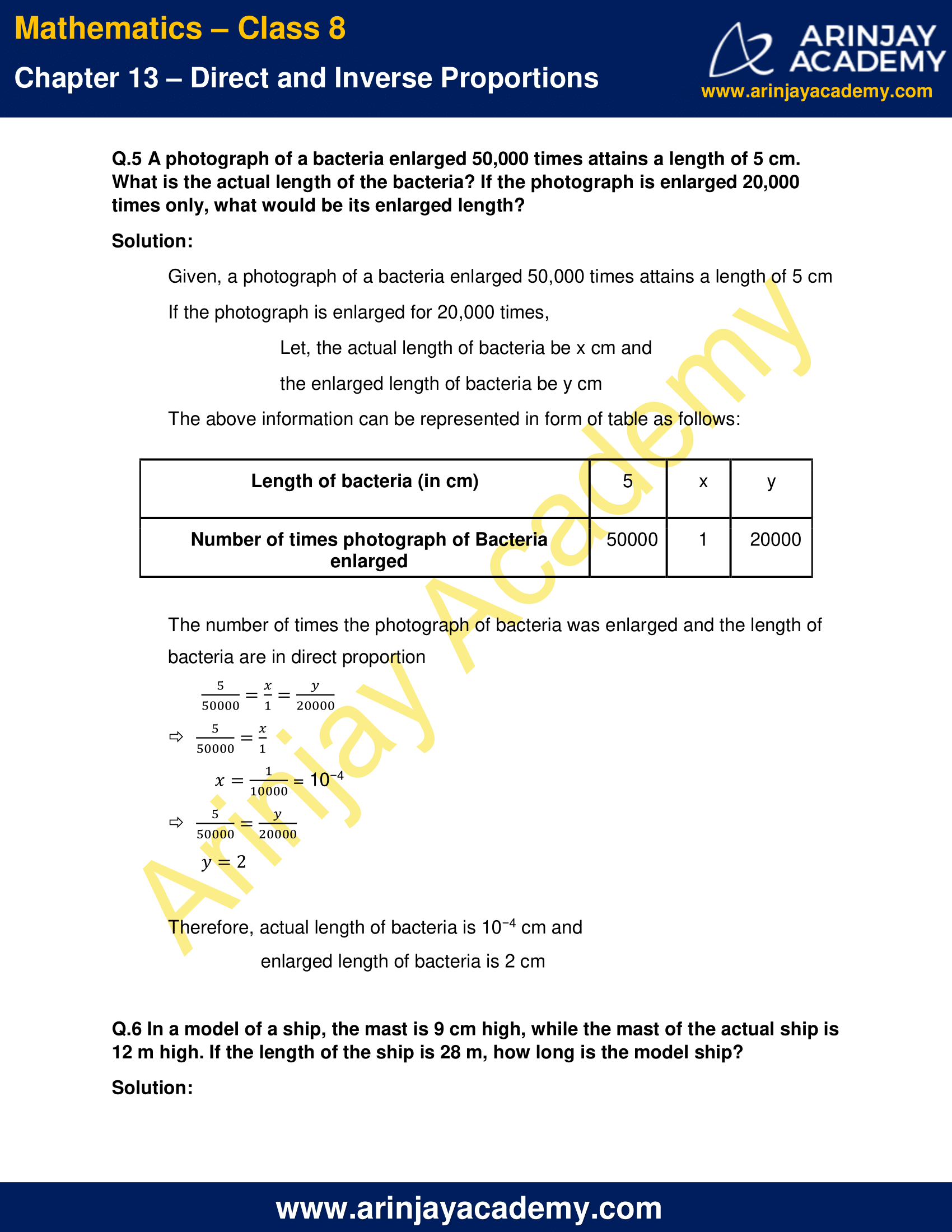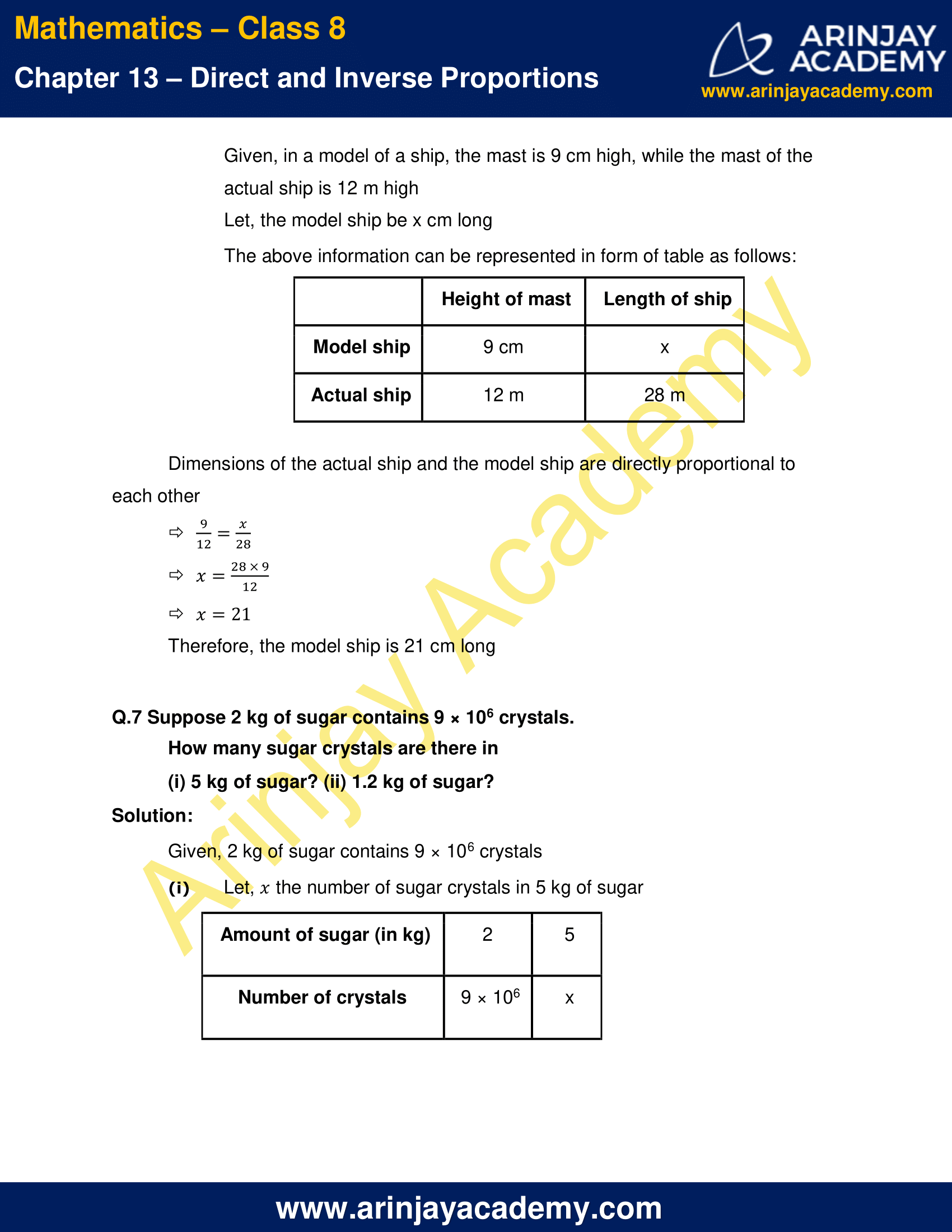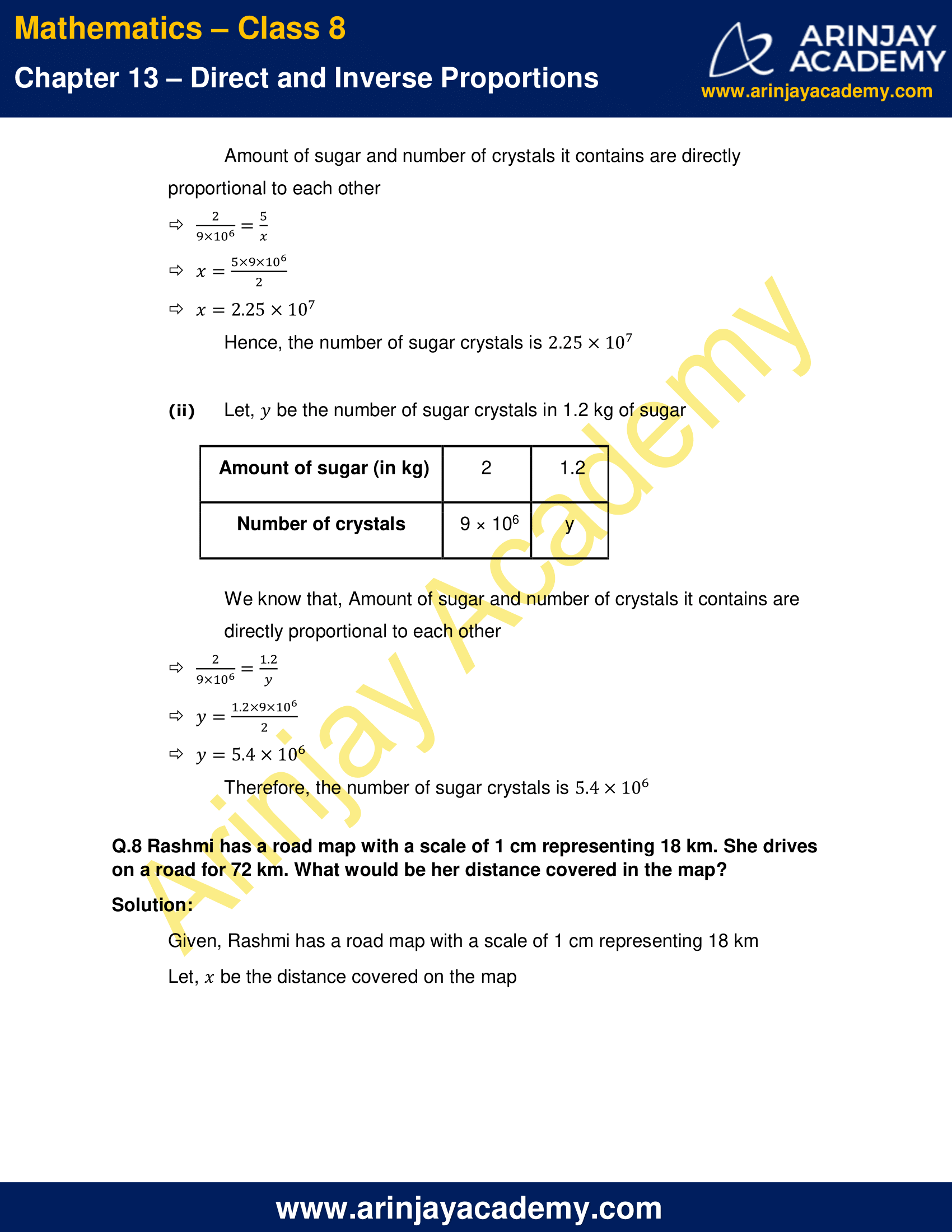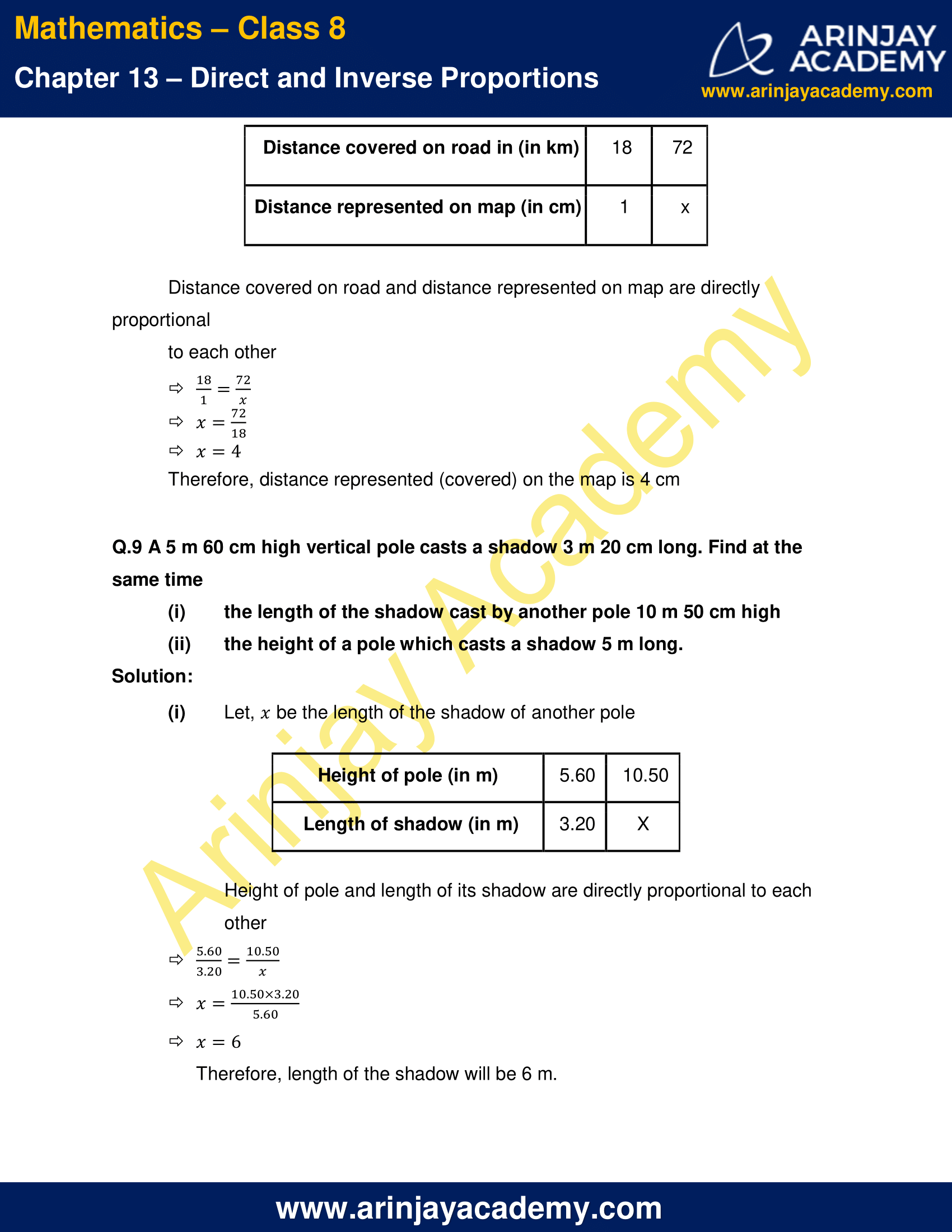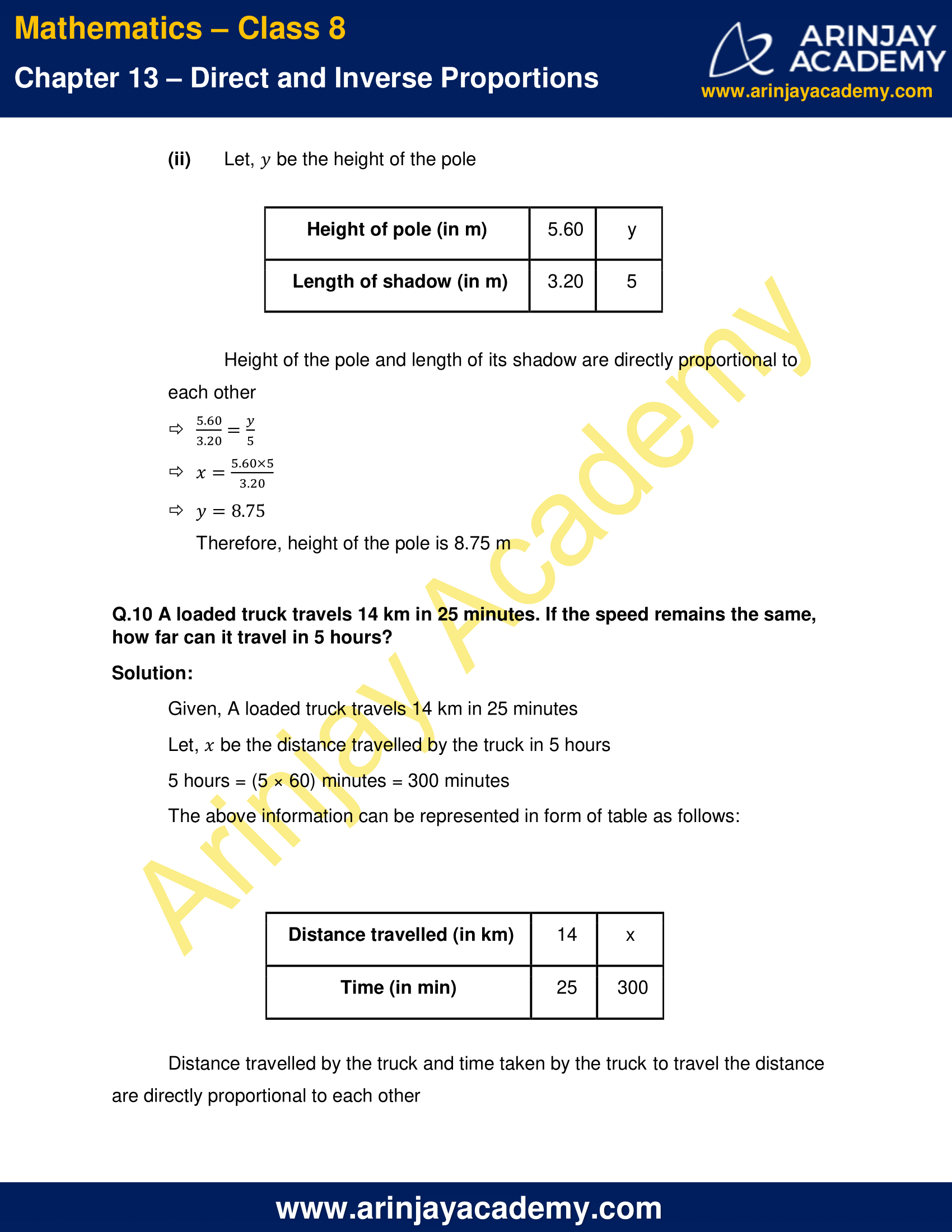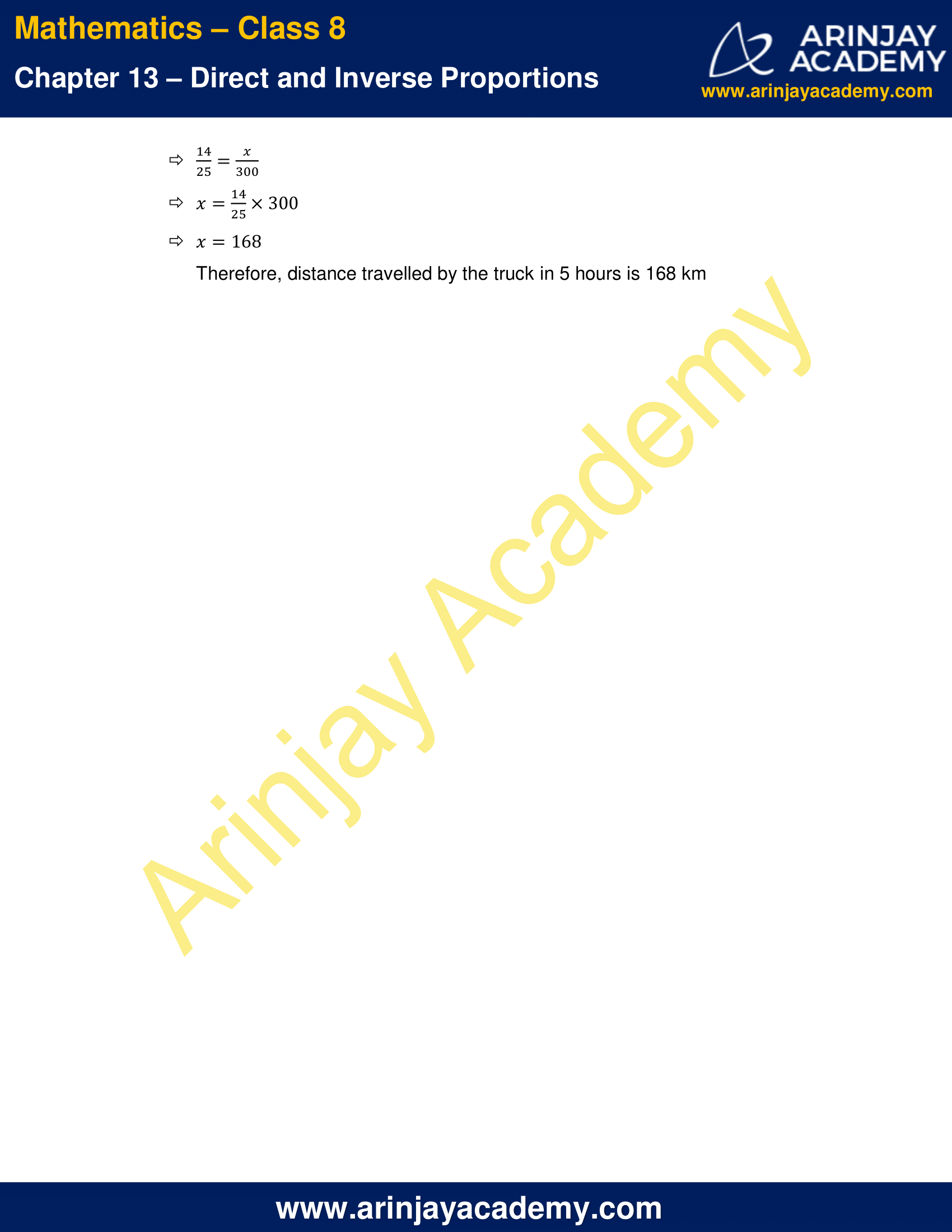NCERT Solutions For Class 8 Maths Chapter 13 Exercise 13.1 – Direct and Inverse Proportions

Q.1 Following are the car parking charges near a railway station up to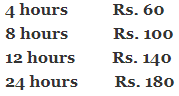Check if the parking charges are in direct proportion to the parking time.

Solution:

We put the given information in the form of a table as shown below: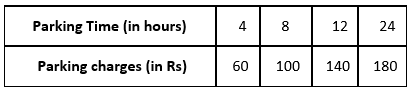The ratio of parking charges to the respective parking time duration:

60/4 = 15
100/8 = 25/2
140/12 = 35/3
180/24 = 15/2

As each ratio is not same, the parking charges are not in a direct proportion to the parking time.

Q.2 A mixture of paint is prepared by mixing 1 part of red pigments with 8 parts of base. In the following table, find the parts of base that need to be added.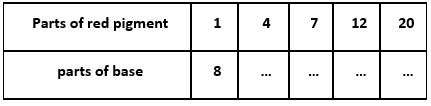Solution:

Given, a mixture of paint is prepared by mixing 1 part of red pigments with 8 parts of base
The parts of base will be in direct proportion to the parts of red pigment
We replace the unknown parts of base with x1, x2, x3 and x4 respectively: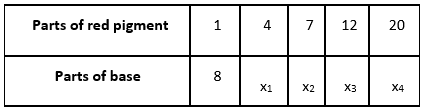According to direct proportion,

x1 /4 = 8/1 => x1 = 32
x2 /7 = 8/1 => x2 = 56
x3 /12 = 8/1 => x3 = 96
x4 /20 = 8/1 => x4 = 160

The table can be completed as: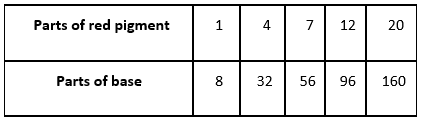Q.3 In Question 2 above, if 1 part of a red pigment requires 75 mL of base, how much red pigment should we mix with 1800 mL of base?

Solution:

Let, the parts of red pigment required to mix with 1800 mL of base be x
The given information can be represented in form of table as follows: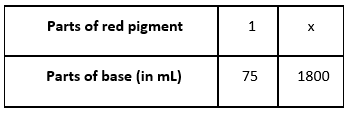The parts of red pigment and the parts of base are in direct proportion

x/1800 = 1/75

x = 1800/75

x = 24

Therefore, 24 parts of red pigments should be mixed with 1800 mL of base

Q.4 A machine in a soft drink factory fills 840 bottles in six hours. How many bottles will it fill in five hours?

Solution:

Given, a machine in a soft drink factory fills 840 bottles in six hours
Let, the number of bottles filled by the machine in five hours be ‘x’

The given information can be represented in form of table as follows: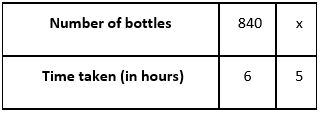Number of bottles and the time taken to fill bottles are in direct proportion
x/5 = 840/6
x = (840/6) × 5
Therefore, 700 bottles will be filled in 5 hours

Q.5 A photograph of a bacteria enlarged 50,000 times attains a length of 5 cm. What is the actual length of the bacteria? If the photograph is enlarged 20,000 times only, what would be its enlarged length?

Solution:

Given, a photograph of a bacteria enlarged 50,000 times attains a length of 5 cm
If the photograph is enlarged for 20,000 times,
Let, the actual length of bacteria be x cm and the enlarged length of bacteria be y cm
The above information can be represented in form of table as follows: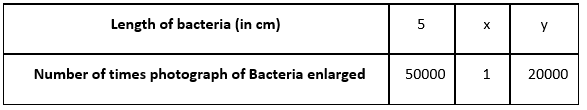The number of times the photograph of bacteria was enlarged and the length of bacteria are in direct proportion
5/50000 = x/1 = y/20000
5/50000 = x/1
x = 1/10000 = 10−4
5/50000 = y/20000
y = 2
Therefore, actual length of bacteria is 10−4 cm and enlarged length of bacteria is 2 cm

Q.6 In a model of a ship, the mast is 9 cm high, while the mast of the actual ship is 12 m high. If the length of the ship is 28 m, how long is the model ship?

Solution:

Given, in a model of a ship, the mast is 9 cm high, while the mast of the actual ship is 12 m high
Let, the model ship be x cm long
The above information can be represented in form of table as follows: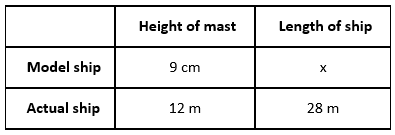Dimensions of the actual ship and the model ship are directly proportional to each other
9/12 = x/28
x = (28×9)/12
x = 21
Therefore, the model ship is 21 cm long

Q.7 Suppose 2 kg of sugar contains 9 × 106 crystals.
How many sugar crystals are there in
(i) 5 kg of sugar?
(ii) 1.2 kg of sugar?

Solution:

Given, 2 kg of sugar contains 9 × 106 crystals
(i) Let,  the number of sugar crystals in 5 kg of sugarAmount of sugar and number of crystals it contains are directly proportional to each other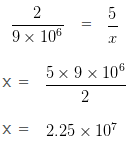(ii) Let, be the number of sugar crystals in 1.2 kg of sugar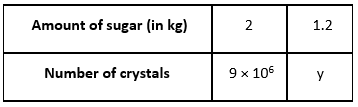We know that, Amount of sugar and number of crystals it contains are directly proportional to each other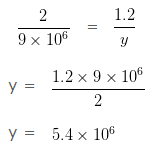Q.8 Rashmi has a road map with a scale of 1 cm representing 18 km. She drives on a road for 72 km. What would be her distance covered in the map?

Solution:

Given, Rashmi has a road map with a scale of 1 cm representing 18 km
Let, ‘x’ be the distance covered on the map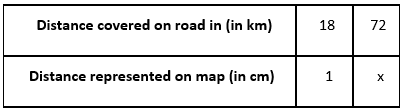Distance covered on road and distance represented on map are directly proportional to each other
18/1 = 72/x
x = 72/18
x = 4
Therefore, distance represented (covered) on the map is 4 cm

Q.9 A 5 m 60 cm high vertical pole casts a shadow 3 m 20 cm long. Find at the same time

(i) the length of the shadow cast by another pole 10 m 50 cm high
(ii) the height of a pole which casts a shadow 5 m long.

Solution:

(i) Let, x be the length of the shadow of another pole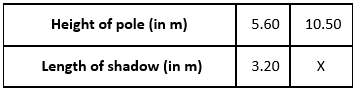5.60/3.20 = 10.50/x
x = (10.50×3.20)/5.60
x = 6

Therefore, length of the shadow will be 6 m.

(ii) Let, y be the height of the pole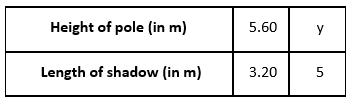Height of the pole and length of its shadow are directly proportional to each other
5.60/3.20 = y/5
x = (5.60×5)/3.20
x = 8.75
Therefore, height of the pole is 8.75 m

Q.10 A loaded truck travels 14 km in 25 minutes. If the speed remains the same, how far can it travel in 5 hours?

Solution:

Given, A loaded truck travels 14 km in 25 minutes
Let, x be the distance travelled by the truck in 5 hours
5 hours = (5 × 60) minutes = 300 minutes
The above information can be represented in form of table as follows: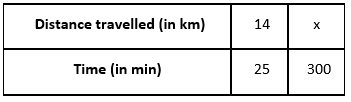Distance travelled by the truck and time taken by the truck to travel the distance are directly proportional to each other
14/25 = x/300
x = (14/25) x 300
x = 168
Therefore, distance travelled by the truck in 5 hours is 168 km

The next Exercise for NCERT Solutions for Class 8 Maths Chapter 13 Exercise 13.2 – Direct and Inverse Proportions can be accessed by clicking here

Download NCERT Solutions For Class 8 Maths Chapter 13 Exercise 13.1 – Direct and Inverse Proportions